﻿ CCSS 2.NBT.5 Worksheets.  Two Digit Addition and Subtraction Within 100 Worksheets.

# Common Core State Standard 2.NBT.5

## Number & Operations in Base Ten

### Use place value understanding and properties of operations to add and subtract.

5. Fluently add and subtract within 100 using strategies based on place value, properties of operations, and/or the relationship between addition and subtraction.

**  The CCSS does not focus on the regrouping algorithm for second grade, but your state/district might include it in your curriculum.  There are regrouping worksheets below.

###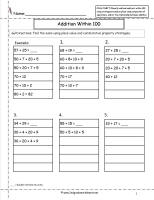Addition Worksheets2345678910

Common Core State Standards: 2.NBT.5
Number & Operations in Base Ten
Use Place Value Understanding and Properties of Operations to Add and Subtract.
Fluently add and subtract within 100 using strategies based on place value, properties of operations, and/or the relationship between addition and subtraction.

Students complete addition problems using place value and commutative.

###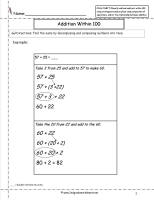Addition Worksheets 23

Common Core State Standards: 2.NBT.5
Number & Operations in Base Ten
Use Place Value Understanding and Properties of Operations to Add and Subtract.
Fluently add and subtract within 100 using strategies based on place value, properties of operations, and/or the relationship between addition and subtraction.

Students complete addition problems by composing tens.

###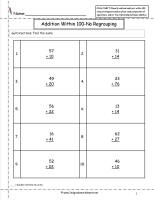Addition Worksheets2345 6789101112131415

Common Core State Standards: 2.NBT.5
Number & Operations in Base Ten
Use Place Value Understanding and Properties of Operations to Add and Subtract.
Fluently add and subtract within 100 using strategies based on place value, properties of operations, and/or the relationship between addition and subtraction.
Students complete addition problems without regrouping.  10 problems.

###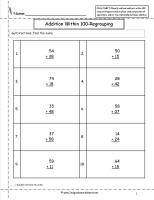Addition Worksheets 2 3 4 5 6 7 89 101112131415

Common Core State Standards: 2.NBT.5
Number & Operations in Base Ten
Use Place Value Understanding and Properties of Operations to Add and Subtract.
Fluently add and subtract within 100 using strategies based on place value, properties of operations, and/or the relationship between addition and subtraction.
Students complete addition problems with regrouping.  10 problems.

###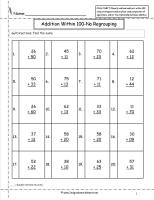Addition Worksheets23456789101112131415

Common Core State Standards: 2.NBT.5
Number & Operations in Base Ten
Use Place Value Understanding and Properties of Operations to Add and Subtract.
Fluently add and subtract within 100 using strategies based on place value, properties of operations, and/or the relationship between addition and subtraction.
Students complete addition problems without regrouping.  20 problems.

###Addition Worksheets23456789101112131415

Common Core State Standards: 2.NBT.5
Number & Operations in Base Ten
Use Place Value Understanding and Properties of Operations to Add and Subtract.
Fluently add and subtract within 100 using strategies based on place value, properties of operations, and/or the relationship between addition and subtraction.
Students complete addition problems with regrouping.  20 problems..

###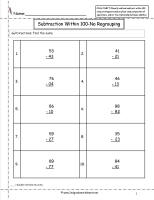Subtraction Worksheets2345678910

Common Core State Standards: 2.NBT.5
Number & Operations in Base Ten
Use Place Value Understanding and Properties of Operations to Add and Subtract.
Fluently add and subtract within 100 using strategies based on place value, properties of operations, and/or the relationship between addition and subtraction.
Students complete subtraction problems without regrouping. 10 problems.

###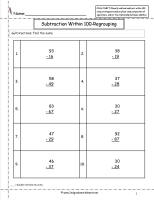Subtraction Worksheets23456 78 9101112131415

Common Core State Standards: 2.NBT.5
Number & Operations in Base Ten
Use Place Value Understanding and Properties of Operations to Add and Subtract.
Fluently add and subtract within 100 using strategies based on place value, properties of operations, and/or the relationship between addition and subtraction.
Students complete subtraction problems with regrouping. 10 problems.

###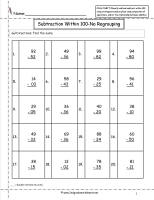Subtraction Worksheets23456789101112131415

Common Core State Standards: 2.NBT.5
Number & Operations in Base Ten
Use Place Value Understanding and Properties of Operations to Add and Subtract.
Fluently add and subtract within 100 using strategies based on place value, properties of operations, and/or the relationship between addition and subtraction.
Students complete subtraction problems without regrouping. 20 problems.

###Subtraction Worksheets234567 891011 12131415

Common Core State Standards: 2.NBT.5
Number & Operations in Base Ten
Use Place Value Understanding and Properties of Operations to Add and Subtract.
Fluently add and subtract within 100 using strategies based on place value, properties of operations, and/or the relationship between addition and subtraction.
Students complete subtraction problems with regrouping. 20 problems.

###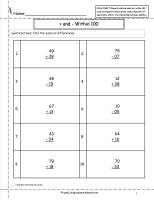Subtraction Worksheets23 4567 891011 121314151617181920

Common Core State Standards: 2.NBT.5
Number & Operations in Base Ten
Use Place Value Understanding and Properties of Operations to Add and Subtract.
Fluently add and subtract within 100 using strategies based on place value, properties of operations, and/or the relationship between addition and subtraction.
Students complete + and - problems with regrouping.  10 problems.

###Subtraction Worksheets2345678910

Common Core State Standards: 2.NBT.5
Number & Operations in Base Ten
Use Place Value Understanding and Properties of Operations to Add and Subtract.
Fluently add and subtract within 100 using strategies based on place value, properties of operations, and/or the relationship between addition and subtraction.
Students complete + and - problems with regrouping.  20 problems.

###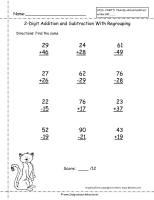Addition and Subtraction Worksheets 1234567 8910

Common Core State Standards: 2.NBT.5
Number & Operations in Base Ten
Use Place Value Understanding and Properties of Operations to Add and Subtract.
Fluently add and subtract within 100 using strategies based on place value, properties of operations, and/or the relationship between addition and subtraction.
Students complete + and - problems with regrouping. 12 problems.